# AP Physics B : Using Ohm's Law

## Example Questions

### Example Question #1 : Using Ohm's Law

A student assembles a circuit made up of a voltage source and two resistors. All three circuit elements are connected in series. If the voltage across the voltage source is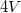and the resistance of the resistors are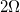and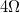respectively, what is current through the second resistor?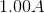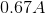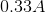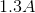Explanation:

Recall that the current through resistors connected in series is the equal for all components. The current through the first resistor and the current through the second resistor should be the same.

The first step in solving involves calculating the equivalent resistance of the circuit. Since the resistors are in series the equivalent resistance of this circuit is the sum of the two resistances.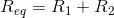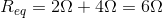Now we can use Ohm’s law to solve for the current.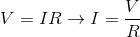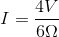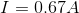### All AP Physics B Resources